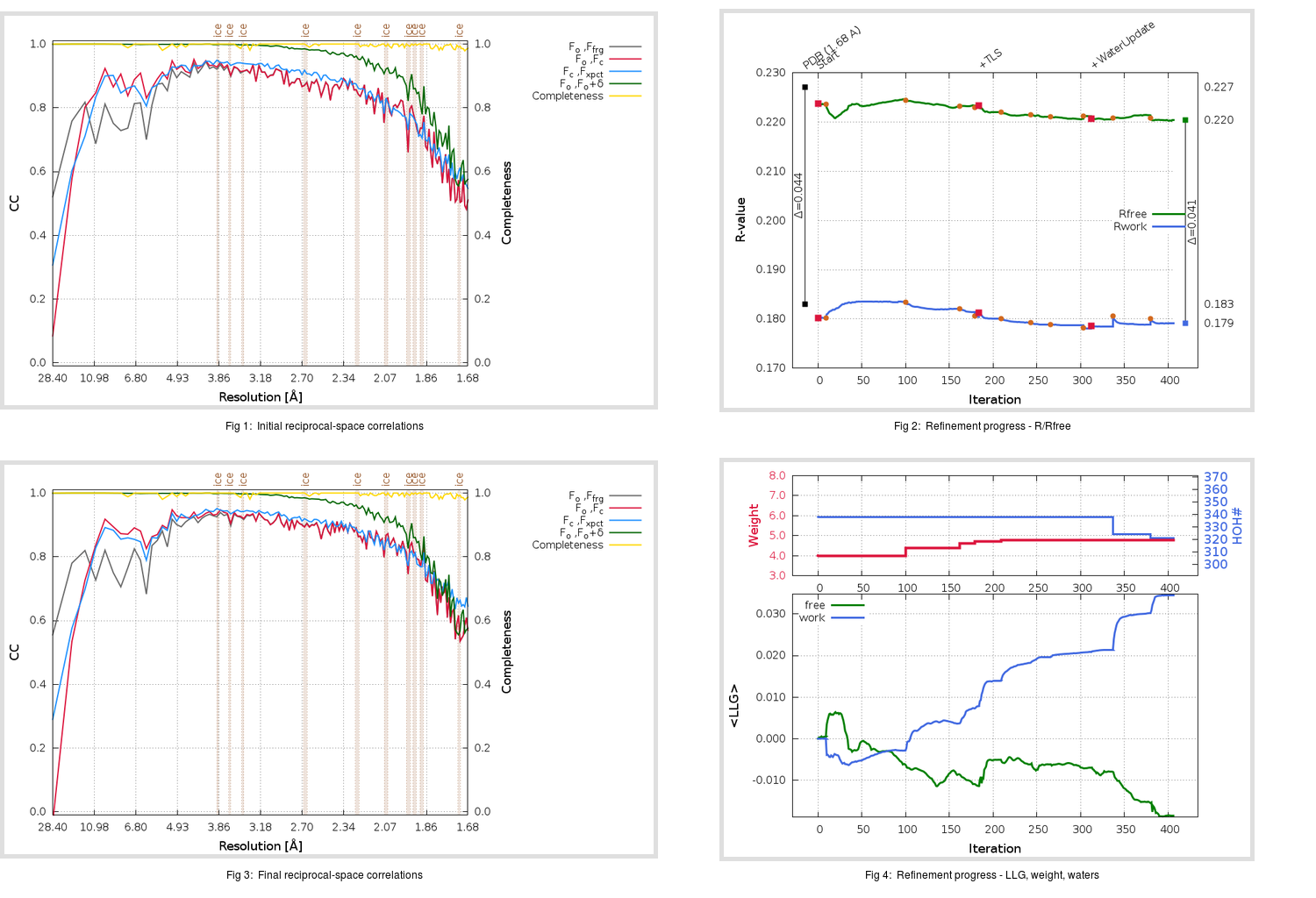Content:

## Deposited

` `
 Date deposited Date data collection Resolution R, Rfree 20200315 20200306 1.68 0.1810 0.2270

Molprobity (CCP4 7.0 version) summary:

```Ramachandran outliers =   0.33 %
favored =  98.01 %
Rotamer outliers      =   0.72 %
C-beta deviations     =     0
Clashscore            =   5.64
RMS(bonds)            =   0.0130
RMS(angles)           =   1.82
MolProbity score      =   1.31
Resolution            =   1.68
R-work                =   0.1810
R-free                =   0.2270
```

```Number of waters      =   338

<B> (all atoms) =   23.34 ( sd =    8.13 ) for       2842 non-hydrogen atoms
<B>   (protein) =   21.99 ( sd =    7.11 ) for       2473 non-hydrogen atoms
<B>     (water) =   32.52 ( sd =    9.11 ) for        338 non-hydrogen atoms
<B>    (others) =   29.48 ( sd =    9.14 ) for         31 non-hydrogen atoms

B min/max       (all non-hydrogen atoms) =    8.21 /   58.85
B min/max   (protein non-hydrogen atoms) =   13.16 /   58.85
B min/max     (water non-hydrogen atoms) =    8.21 /   54.91
B min/max     (other non-hydrogen atoms) =   23.72 /   38.59
```

## BUSTER (re-)refinement

` `

Molprobity (CCP4 7.0 version) summary:

```Ramachandran outliers =   0.33 %
favored =  98.01 %
Rotamer outliers      =   1.09 %
C-beta deviations     =     0
Clashscore            =   4.43
RMS(bonds)            =   0.0113
RMS(angles)           =   1.61
MolProbity score      =   1.25
Resolution            =   1.68
R-work                =   0.1791
R-free                =   0.2204
```

```Number of waters      =   321

<B> (all atoms) =   23.98 ( sd =    8.23 ) for       2825 non-hydrogen atoms
<B>   (protein) =   22.42 ( sd =    6.57 ) for       2473 non-hydrogen atoms
<B>     (water) =   35.19 ( sd =   10.31 ) for        321 non-hydrogen atoms
<B>    (others) =   29.35 ( sd =   10.81 ) for         31 non-hydrogen atoms

B min/max       (all non-hydrogen atoms) =    8.11 /   66.52
B min/max   (protein non-hydrogen atoms) =    8.27 /   53.59
B min/max     (water non-hydrogen atoms) =    8.11 /   66.52
B min/max     (other non-hydrogen atoms) =   20.14 /   41.84
```

Refinement progression:Results:

` `
 File Remark 5RFZ_aB_refine.01_03_refine.pdb.gz exact refinement commands are in header 5RFZ_aB_refine.01_03_refine.mtz.gz including original deposited data and several re-refinement map coefficients 5RFZ_aB_refine.01_03_BUSTER_model.cif.gz including any non-standard compound restraints 5RFZ_aB_refine.01_03_BUSTER_refln.cif.gz